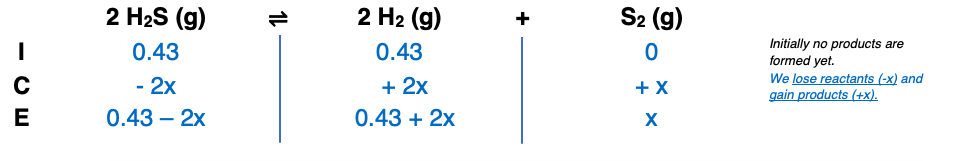# Problem: Consider the reaction for the decomposition of hydrogen disulfide: 2H2S(g) ⇌ 2H2(g) + S2(g), Kc = 1.67 x 10–7 at 800 oC. A 0.500 L reaction vessel initially contains 0.125 mol of H2S and 0.125 mol of H2 at 800 oC. Find the equilibrium concentration of [S2].

###### FREE Expert Solution

= 0.43 M

= 0.43 M90% (142 ratings)###### Problem Details

Consider the reaction for the decomposition of hydrogen disulfide: 2H2S(g) ⇌ 2H2(g) + S2(g), Kc = 1.67 x 10–7 at 800 oC. A 0.500 L reaction vessel initially contains 0.125 mol of H2S and 0.125 mol of H2 at 800 oC. Find the equilibrium concentration of [S2].

Frequently Asked Questions

What scientific concept do you need to know in order to solve this problem?

Our tutors have indicated that to solve this problem you will need to apply the ICE Chart concept. You can view video lessons to learn ICE Chart. Or if you need more ICE Chart practice, you can also practice ICE Chart practice problems.

What professor is this problem relevant for?

Based on our data, we think this problem is relevant for Professor Griffith's class at UMD.